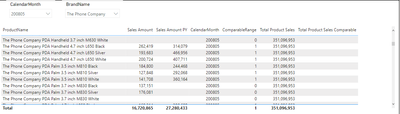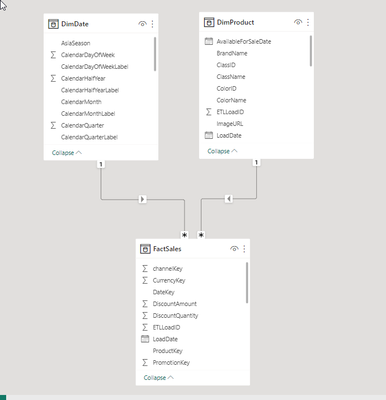cancel
Showing results for
Did you mean:Helper I

## How to use a measure as a filter in another measure ?

Hello community!

I am trying to create a measure that calculates the total product sales for a specfic month only for does products that has been sold the same period last year.

The first thing I did was to create a measure to calculate the Sales Amount for previous year:
Sales Amount PY =
CALCULATE([Sales Amount],SAMEPERIODLASTYEAR(DimDate[Datekey]))

The second thing I did was to create a Comparable range flag as measure:

ComparableRange = IF(FactSales[Sales Amount] = BLANK() || FactSales[Sales Amount PY] = BLANK(),0,1)

Third step I created a measure to calculate the total product sales:
Total Product Sales =
CALCULATE(FactSales[Sales Amount],ALL(DimProduct))

The final step I want to calculate the total product sales only for does products being comparable.
I tried this solution but not getting it to work, it is only returning blank:
Total Product Sales Comparable =
var FilteredTable = FILTER(CompRangeTable,[@CompRange] = 1)
return
CALCULATE(FactSales[Sales Amount],ALL(DimProduct),FilteredTable)I also tried this solution but still getting blanks:
Total Product Sales Comparable =
var FilteredTable = FILTER(FactSales, [Sales Amount PY]*[Sales Amount]+0<>0)
return
CALCULATE([Sales Amount],ALL(DimProduct),FilteredTable)

To demonstrate my problem I have used the ContosoRetailDW sample database with a simple star scheme consisting in the tables "FactSales", "DimDate" and "DimProduct"Thanks in advanced for any help.

1 ACCEPTED SOLUTIONHelper I

I re-wrote the solution to the following:

sumx(filter(All(DimProduct[ProductName]),[Sales Amount PY]*[Sales Amount]+0<>0),FactSales[Sales Amount])
Which produces the desired result and with less code.
5 REPLIES 5Super User

A measure is returning a scalar value. A filter expects a table. Rewrite your second measure to incorporate the same logic as you did in the first.Helper I

I adjusted the measure to create the CompRangeTable based on DimProduct instead of the fact table:

Total Product Sales Comparable v1 =
var FilteredTable = FILTER(CompRangeTable,[@CompRange] = 1)
return
CALCULATE(FactSales[Sales Amount],ALL(DimProduct),FilteredTable)

And it is able to display the Sales Amount that is comparable. The only issue is that is seems to ignore the ALL(DimProduct) in the return statement. So it only returns the same as the "Sales Amount"It should return 13,811,177 on each row which is the total:Super User

Use a step by step approach with variables and use EVALUATEANDLOG to ensure each step is producing the desired result.Helper I

I re-wrote the solution to the following:

sumx(filter(All(DimProduct[ProductName]),[Sales Amount PY]*[Sales Amount]+0<>0),FactSales[Sales Amount])
Which produces the desired result and with less code.Helper I

Thank you for you respons Ibendlin!
When you say the second measure, do you mean I should adjust this one ?

Total Product Sales Comparable =
var FilteredTable = FILTER(FactSales, [Sales Amount PY]*[Sales Amount]+0<>0)
return
CALCULATE([Sales Amount],ALL(DimProduct),FilteredTable)